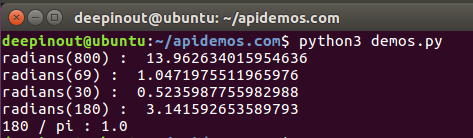1. 角度转换为弧度公式：弧度=角度÷180×π
2. 弧度转换为角度公式： 角度=弧度×180÷π

import math

math.radians(x)

• x : 一个角度数值，默认单位是角度 °

#!/usr/bin/python3
import math

print("180 / pi : ", end ="")
print (math.radians(180 / math.pi))• 微信订阅
• 回顶